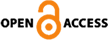### Current Issue

HOMECurrent Issue Current Issue

Asian Review of Financial Research Vol.34 No.3 pp.65-91 https://www.doi.org/10.37197/ARFR.2021.34.3.3
The Relationship between Non-interest Revenue and Profitability in Korean Banks
Key Words : Non-interest revenue,Profitability,Korean banks,Non-linear relationship,Revenue diversification

### Abstract

In the current low-interest rates environment, Korean banks' profitability is expected to weaken significantly because of the large proportion of interest revenue in total revenue. For instance, Korean banks' interest revenue accounted for 84 percent of total revenues in 2019. As a results, Korean banks need to accelerate their strategy for revenue diversification, such as increasing non-interest revenue in total revenue. In general, revenue diversification, such as increasing a non-interest revenue, is expected to stabilize or improve banks' profitability. However, previous empirical studies show inconsistent results on the relationship between non-interest revenue and total profitability in banks. For instance, Smith et al. (2003) argue that banks' profitability is stabilized by diversifying revenue if the non-interest revenue increases. In contrast, De Young and Roland (2001), Stiroh and Rumble (2006) show that the high volatility of non-interest revenue offsets revenue diversification. Stiroh (2004) argues that the increase in non-interest income does not lead to risk diversification because of the the high correlation between interest revenue and non-interest revenue in analysis for U.S. banks. Thus, unlike previous studies, the paper analyzes the relationship between the proportion of non-interest revenue and profitability in Korean banks using the U-shaped test method of Lind and Mehlum (2010) to determine a nonlinear relationship between the proportion of non-interest revenue and profitability. Empirical results using the financial statements of 15 Korean banks from 2008 to 2019 show that Korean banks' profitability and the proportion of non-interest revenue have an inverted U-shaped relationship. Here, the maximum point of an inverted U-shape represents the optimal proportion level of non-interest revenue that generates the highest banks' profitability. It means that profitability improves as the proportion of non-interest revenue increases until the optimal proportion level of non-interest revenue. However, exceeded an optimal proportion level of non-interest revenue, the profitability decreases as the proportion of non-interest revenue increases. This inverted U-shape relationship is interpreted as follows. In a positive relationship between non-interest revenue and profitability, an increase in non-interest revenue obtains an additional profit channel and generates a revenue diversification effect. On the other hand, when non-interest revenue and profitability have a negative relationship, the increasing non-interest revenue causes a reduction in the opportunity profit of interest revenue and amplifies revenue uncertainty by the high volatility of non-interest revenue. The results indicate that Korean banks' excessive concentration of the management capabilities on non-interest operations may decrease the total profitability. In particular, the study also has found that Korean banks have a high correlation between commission revenue, which accounts for the largest proportion of non-interest revenue, and interest revenue. Thus, the result implies that Korean banks' increasing non-interest revenue strategy, although it increases non-interest revenue, has inefficient to achieve revenue diversification effects because of the high correlation between interest revenue and non-interest revenue. For instance, Korean banks often sell products based on non-interest to customers who buy products based on interest; this sales strategy weak the risk diversification effects by overlapping the customer groups between interest and non-interest products. This paper also conducts an empirical analysis by dividing banks into commercial and regional banks. The results show that commercial banks' profitability and the proportion of non-interest revenue have an inverted U-shape relationship. We also empirical test for commercial banks by classifying the observation based on the optimal proportion of non-interest revenue. The results show that the commercial bank's relationship between the proportion of non-interest revenue and profitability is significantly positive in the case of below the optimal proportion level. On the other hand, the commercial bank's relationship between non-interest revenue and profitability is insignificantly negative in the case of above the optimal proportion level, which is attributable to a small number of samples for banks with a proportion of non-interest revenue above the optimal proportion level. That is, the result implies the Korean banks' high reliance on interest revenue. Similarly, local banks have statistically only positive relationships between non-interest revenue and profitability due to the deficient proportion of non-interest revenue. In the current low-interest rate environment, it is inevitable for Korean banks to accelerate expanding non-interest revenue. However, as the results in this paper, excessive non-interest revenue expansion strategies may occur a negative impact on profitability according to an inverted U-shaped relationship between the proportion of non-interest revenue and profitability. Thus, Korean banks should first set up sophisticated and systematic strategies for expanding non-interest revenue to achieve an advantage of increasing non-interest revenue. To do so, banks need to differentiate their sale strategy by customer characteristics and develop their risk management capabilities to manage volatility in non-interest revenue. In addition, it is necessary to derive the optimal proportion of non-interest income for the individual bank by considering the characteristics of an individual bank in the future study.
LIST
Export citation